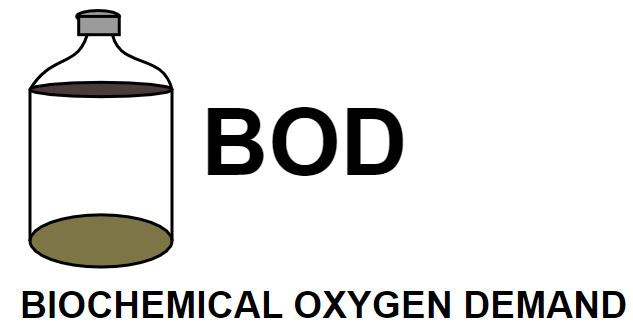top of page
Search
• APSEd

# Biochemical Oxygen Demand (BOD) | BOD Equation & Solved Example

Updated: Dec 10, 2021

Biochemical Oxygen Demand, BOD is defined as the oxygen required by aerobic microorganisms to oxidise the biodegradable organic matter. In other words, the BOD indicates the amount of oxygen that bacteria and other microorganisms consume in a water sample during a specific period at a constant standard temperature (20 degrees celsius) to degrade the water contents aerobically.

BOD is thus an indirect measure of the sum of all biodegradable organic substances in the water. This also indicates how much dissolved oxygen (milligram per litre) is needed in a given time for the biological degradation of the organic wastewater constituents.Some oxygen is always dissolved in water and it is known as dissolved oxygen (DO). DO is necessary for the survival of aquatic life and a minimum of 4 PPM of dissolved oxygen is required for the survival of aquatic life. In the case of wastewater, organic matter present requires the DO to decompose them.

Microorganisms that decompose the organic matter in the presence of oxygen are known as aerobic bacteria. BOD is defined as the oxygen required by aerobic microorganisms to oxidise the biodegradable organic matter.

It can be used for finding the quantity of oxygen required to stabilise the biodegradable organic matter. It helps us in deciding the size of the treatment units of the wastewater treatment plant. It gives us an idea of the efficiency of the process i.e. how much BOD is being removed. Other than that we can find the strength of sewage.

We can further classify organic matters into two groups:

1. Carbonaceous matter: First Stage BOD

2. Nitrogenous matter: Second Stage BOD

Total BOD is the summation of the above two.

## How to find the BOD?

The standard method used in the laboratory to find the BOD of a sample is the 'Dilution Method'. It is a time taking process, in 5 days 60% -70% of the organic matter is decomposed. In 20 days 95%-99% of the organic matter is oxidised. The water sample is diluted with aerated water and the initial DO is found, then it is incubated for 5 days at 20 Degrees Celsius. After these 5 days, we again find the DO which is now known as the final DO.

BOD₅ at 20 Degrees Celsius = (Initial DO - Final DO) x Dilution Factor

The Dilution Factor is defined as the ratio of Volume of diluted sample to Volume of the undiluted sample.

Dilution Factor, DF = Diluted Sample Volume/Undiluted Sample Volume

## Formulation of BOD Equation

To derive the BOD equation, Oxygen Equivalent of Organic Matter Present is plotted against Time. 'Oxygen Equivalent of Organic Matter Present' is nothing but the BOD remaining.The rate of change of BOD directly varies with BOD remaining at that point in time (Lt)

So, dLt/dt ∝ Lt

Integrating both the sides from interval Lo to Lt in time interval 0 to t,

Lt = Lo x e^(-kt)

BOD Consumed, Yt=Lo-Lt

At any time, Yt = Lo - Lo x e^(-kt)

Yt = Lo[1-e^(-kt)]

If we intend to find out the solution in base 10 instead of base e, then

Lt = Lo x 10^(-k't), Where k' = k/2.303

Here the K' is also known as the deoxygenation Constant, which depends upon the temperature. The formulas given here are in at a standard temperature of 20 Degrees Celsius. To convert to some other Temperature,

K' (T Degree Cel.) = K' (20 Degree Cel.) x [1.047] ^(T-20)

To find the ultimate BOD, that happens when t tends to infinity.

So, Ultimate BOD = Lo

To understand these formulas more properly, I have a solved example for you. Do check it out.

## Solve this one!

BOD is a very important topic asked in GATE. Solve this previous year question to get an idea of the kind of question that gets asked.

Thank you for going through the blog. See you soon in the next one. Till then, take care!

See All
bottom of page